# Gold Price Prediction Using Machine Learning In Python

Is it possible to predict where the Gold price is headed?

Yes, let’s use machine learning regression techniques to predict the price of one of the most important precious metal, the Gold.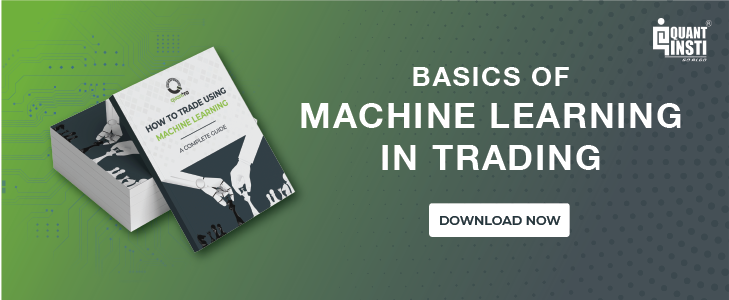We will create a machine learning linear regression model that takes information from the past Gold ETF (GLD) prices and returns a prediction of the Gold ETF price the next day.

GLD is the largest ETF to invest directly in physical gold. (source: http://www.etf.com/GLD)

We will cover the following topics in our journey to predict gold prices using machine learning in python

## Import the libraries and read the Gold ETF data

First things first: import all the necessary libraries which are required to implement this strategy.

Then, we read the past 12 years of daily Gold ETF price data and store it in Df. We remove the columns which are not relevant and drop NaN values using dropna() function. Then, we plot the Gold ETF close price.

Output: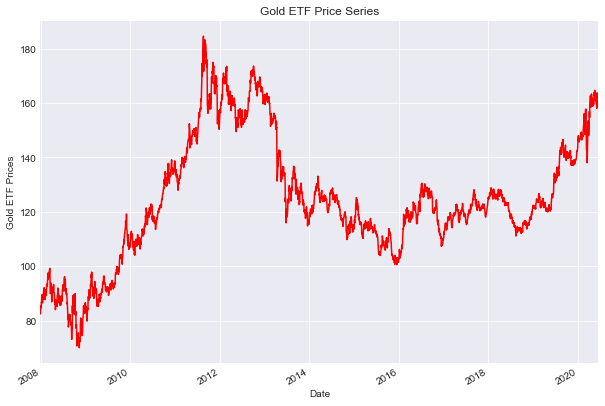## Define explanatory variables

An explanatory variable is a variable that is manipulated to determine the value of the Gold ETF price the next day. Simply, they are the features which we want to use to predict the Gold ETF price. The explanatory variables in this strategy are the moving averages for past 3 days and 9 days. We drop the NaN values using dropna() function and store the feature variables in X.

However, you can add more variables to X which you think are useful to predict the prices of the Gold ETF. These variables can be technical indicators, the price of another ETF such as Gold miners ETF (GDX) or Oil ETF (USO), or US economic data.

## Define dependent variable

Similarly, the dependent variable depends on the values of the explanatory variables. Simply put, it is the Gold ETF price which we are trying to predict. We store the Gold ETF price in y.

## Split the data into train and test dataset

In this step, we split the predictors and output data into train and test data. The training data is used to create the linear regression model, by pairing the input with expected output. The test data is used to estimate how well the model has been trained.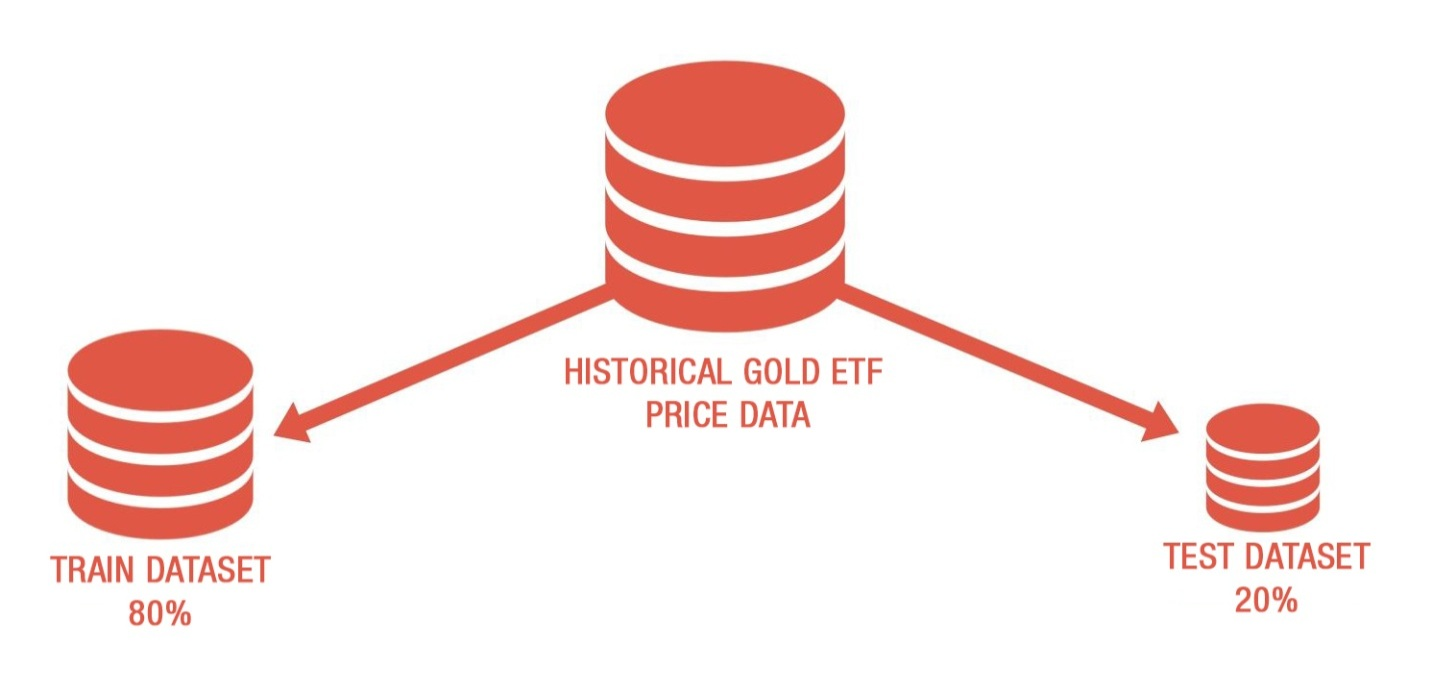1. First 80% of the data is used for training and remaining data for testing
2. X_train & y_train are training dataset
3. X_test & y_test are test dataset

## Create a linear regression model

We will now create a linear regression model. But, what is linear regression?

If we try to capture a mathematical relationship between ‘x’ and ‘y’ variables that “best” explains the observed values of ‘y’ in terms of observed values of ‘x’ by fitting a line through a scatter plots then such an equation between x and y is called linear regression analysis.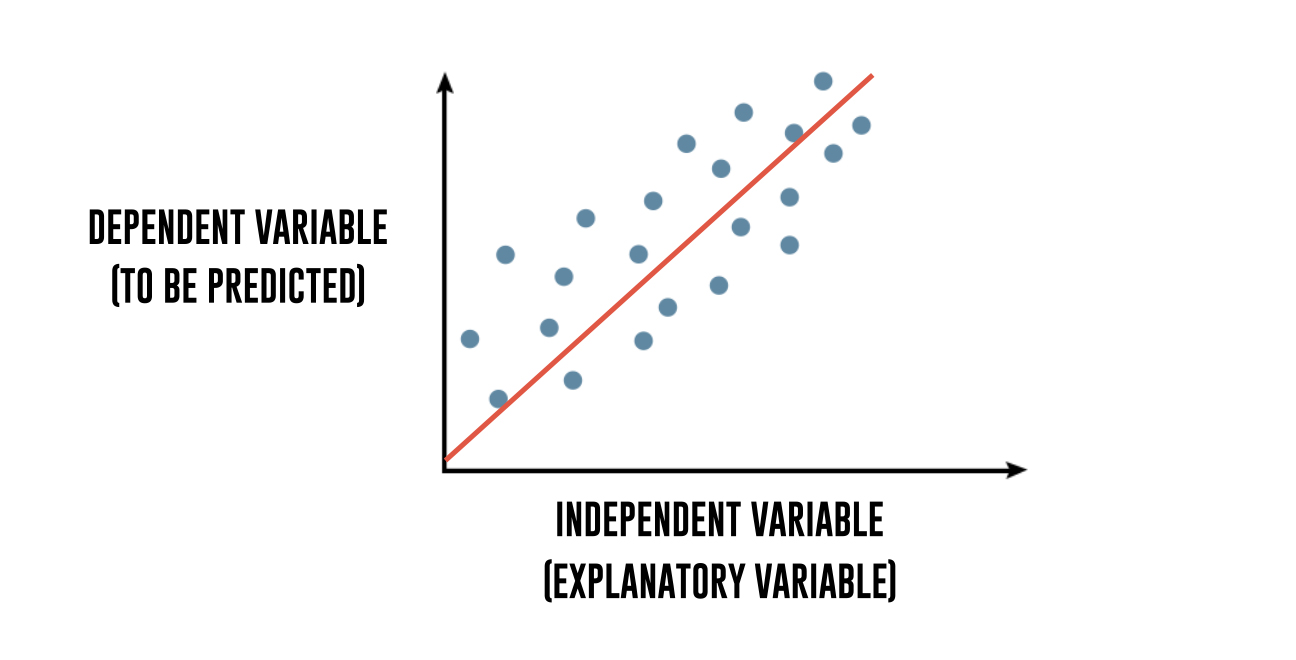To break it down further, regression explains the variation in a dependent variable in terms of independent variables. The dependent variable - ‘y’ is the variable that you want to predict. The independent variables - ‘x’ are the explanatory variables that you use to predict the dependent variable. The following regression equation describes that relation:

Y = m1 * X1 + m2 * X2 + C
Gold ETF price = m1 * 3 days moving average + m2 * 15 days moving average + c

Then we use the fit method to fit the independent and dependent variables (x’s and y’s) to generate coefficient and constant for regression.

Output:

Linear Regression model

Gold ETF Price (y) = 1.20 * 3 Days Moving Average (x1) + -0.21 * 9 Days Moving Average (x2) + 0.43 (constant)

## Predict the Gold ETF prices

Now, it’s time to check if the model works in the test dataset. We predict the Gold ETF prices using the linear model created using the train dataset. The predict method finds the Gold ETF price (y) for the given explanatory variable X.

Output: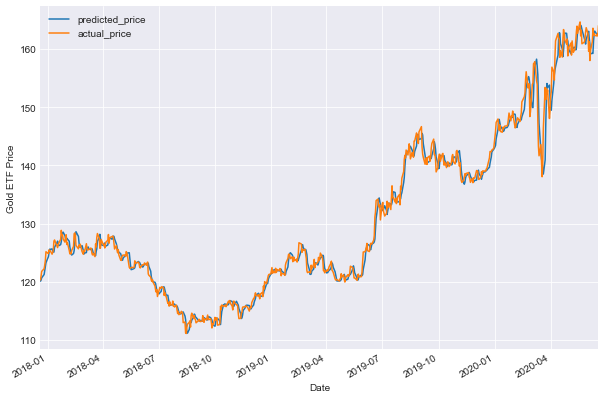The graph shows the predicted and actual price of the Gold ETF.

Now, let’s compute the goodness of the fit using the score() function.

Output:

98.86

As it can be seen, the R-squared of the model is 98.86%. R-squared is always between 0 and 100%. A score close to 100% indicates that the model explains the Gold ETF prices well.

## Plotting cumulative returns

Let’s calculate the cumulative returns of this strategy to analyse its performance.
1. The steps to calculate the cumulative returns are as follows:
2. Generate daily percentage change of gold price
3. Create a buy trading signal represented by “1” when the next day’s predicted price is more than the current day predicted price. No position is taken otherwise
4. Calculate the strategy returns by multiplying the daily percentage change with the trading signal.
5. Finally, we will plot the cumulative returns graph

The output is given below: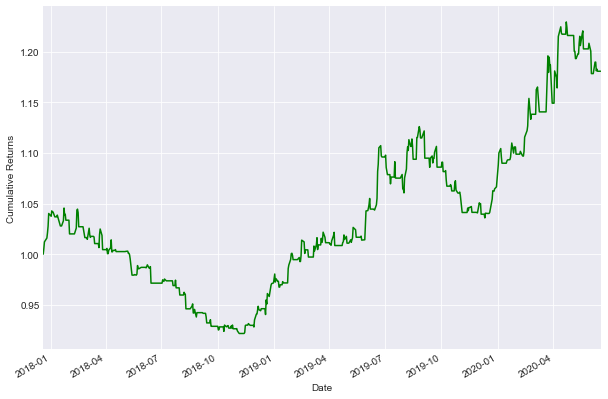We will also calculate the Sharpe ratio.

The output is given below:

'Sharpe Ratio 0.75'

## How to use this model to predict daily moves?

You can use the following code to predict the gold prices and give a trading signal whether we should buy GLD or take no position.

The output is as shown below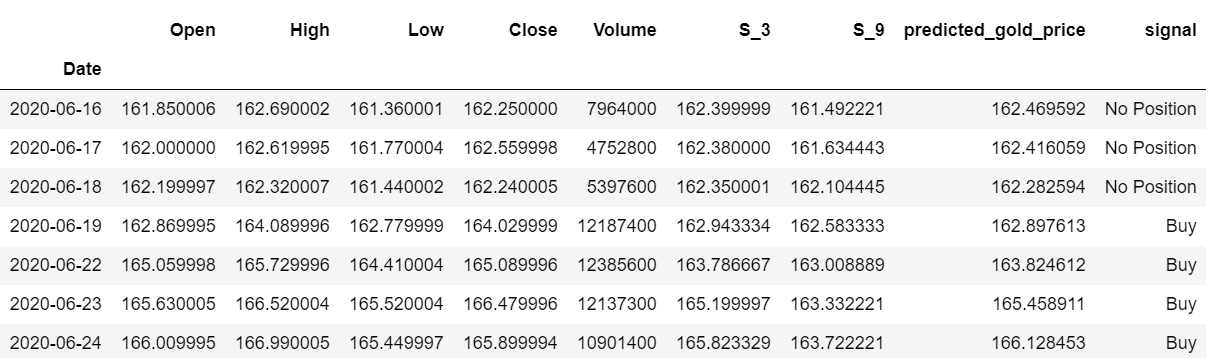Congrats! You just learned a fundamental yet strong machine learning technique. Thanks for reading!